# Things with silly names

Katie Steckles doesn’t understand why any mathematical phenomenon would ever have a not-silly nameWhen I talk about maths research, one thing I often take care to point out to budding mathematicians is to bear in mind just how cool it is that you could be the first person to discover a new thing, or prove an interesting theorem that nobody else has managed to prove before. And while the sheer joy of mathematical discovery and solving the puzzle should be motivation in itself for the future generation of mathematicians, I also make it very clear that it’s often the case that whoever discovers a thing also gets to pick a name for it.

You could take the boring option and name it after yourself (assuming your name is unusual enough that people would know it was actually you—who knows which Bernoulli was responsible for what?). But a much better option is to name your mathematical concept, object or theorem after something amusing, strange or otherwise silly.

In that spirit, here’s my roundup of mathematical things with silly names—you may have your own favourites, but these are a few I’ve been being amused by recently.

## Hedgehog (geometry)

The joy of a Wikipedia page with ‘(geometry)’ in the title is that it usually means there’s something that shares a name with a non-geometrical thing, making it necessary to disambiguate. In which case, hedgehog is the perfect name for this object and nobody can persuade me otherwise.A hedgehog

A hedgehog (in geometry) is a curve in the plane with a particular property. You can consider a curve as being defined by an envelope of lines. A nice example is the curve created by joining points on two axes in pairs, creating a series of lines that gradually change slope, like in the image to the right.

Here the axes range from $0$ to $10$, and a point $p$ on one axis is connected to $11-p$ on the other. The resulting curve is a parabolic curve, and the actual curve itself is referred to as the envelope of the set of straight lines: a curve which is tangent to some member of the family of curves at every point.

In order for such a curve to be a hedgehog—in particular, a ‘sufficiently well-behaved hedgehog’—the curve has to have one tangent line in each oriented direction. That is, for every possible direction you could draw a line pointing in on a 2D plane, there’s some part of the curve that’s pointing in that direction.

Annoyingly, this leads you to the conclusion that a boring circle shape is actually a hedgehog (as in fact is any convex curve—one that doesn’t turn inward at any point). But it’s not the only hedgehog! And the more interesting ones are good fun.

For example, the star shape made by joining four of the parabola we saw earlier is called an astroid, and is an example of a non-convex hedgehog. A similar shape called a deltoid can be made by tracing a point on a circle that’s rolling around the inside of a larger circle with three times the radius—and this is an example of a projective hedgehog.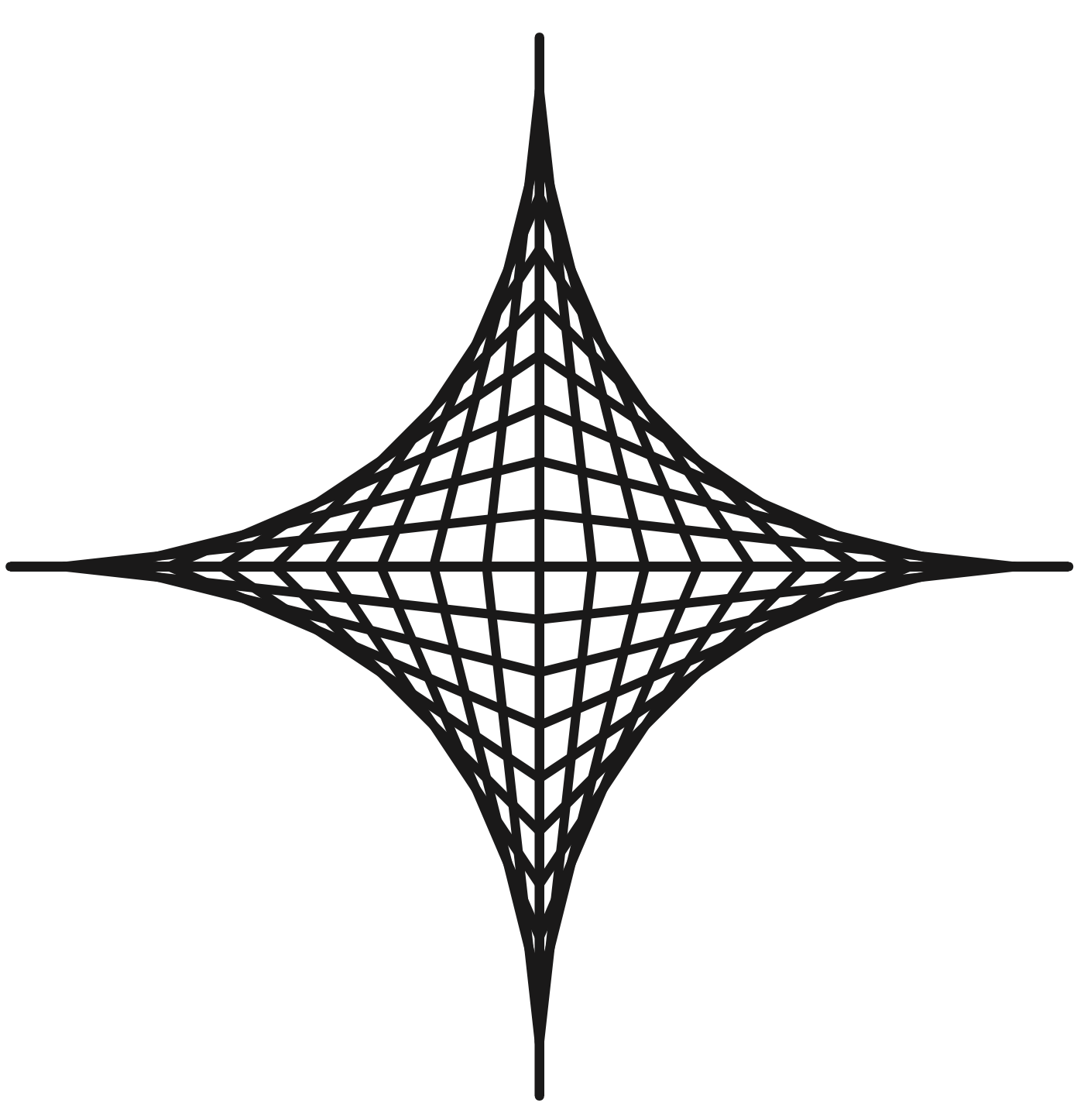An astroid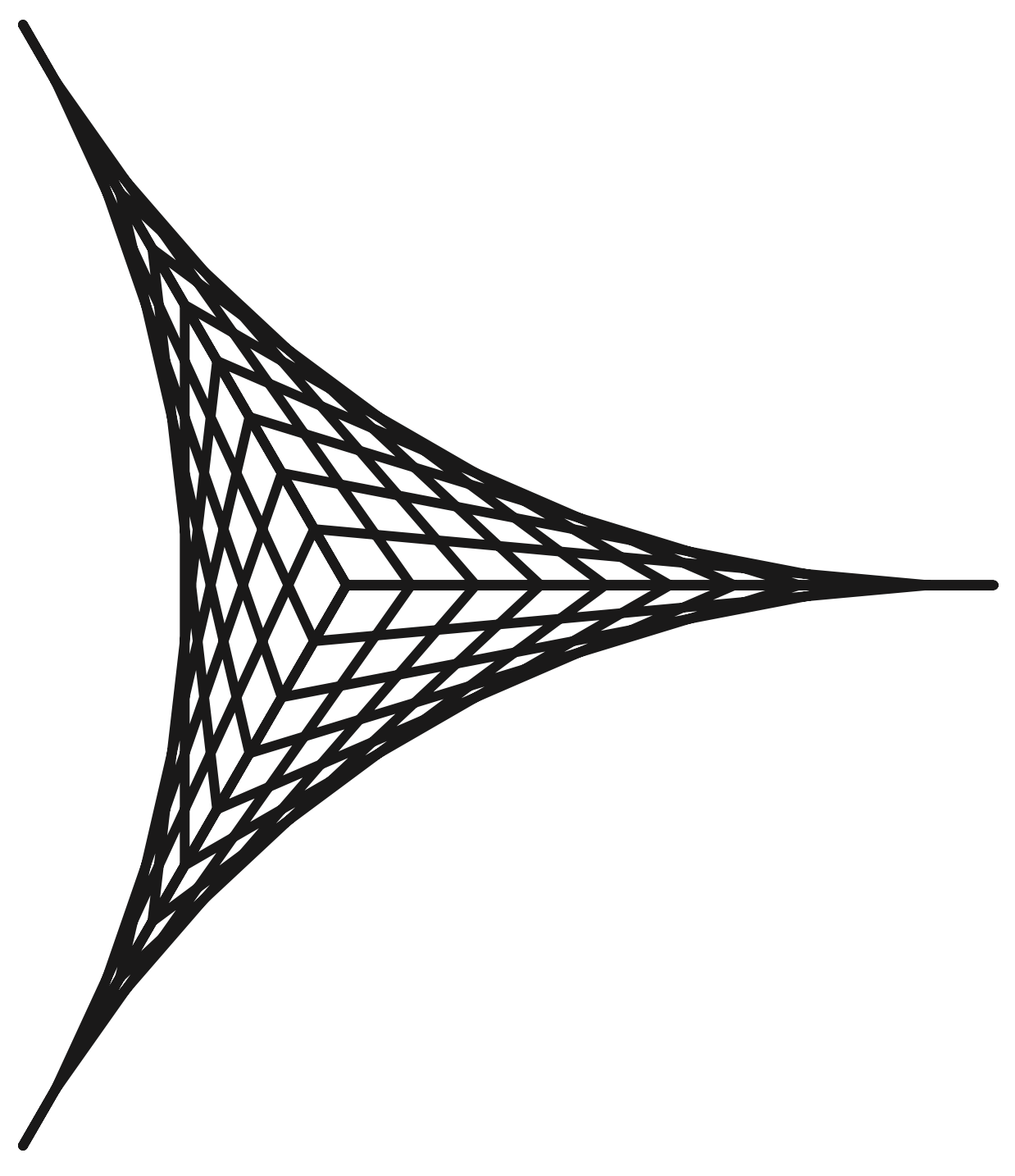A deltoid

Most of the joy of mathematical hedgehogs is in the excellent compound phrases you can make. We can also define the middle hedgehog of a convex shape, and a hypocycloid, made by rolling a general smaller circle around inside a larger one, can form a self-crossing hedgehog (making me think of the road safety adverts from the late 90s).

## The ham sandwich theorem

It’s even better when the object a theorem is named after is something as mundane as a ham sandwich. This theorem is also from geometry, and concerns the possibility of cutting objects in half.

Imagine you have a cake sitting on a table, and you’re looking at it from above (so you’re effectively considering a two-dimensional cake, assuming the cake is of uniform thickness). If you wanted to share the cake with someone else, you could cut it in half using a straight line and have the same amount each. If you had two cakes sitting on the same table, you could also make a single straight cut that cuts both cakes in half exactly. But once you introduce a third cake, you can’t guarantee this will be possible: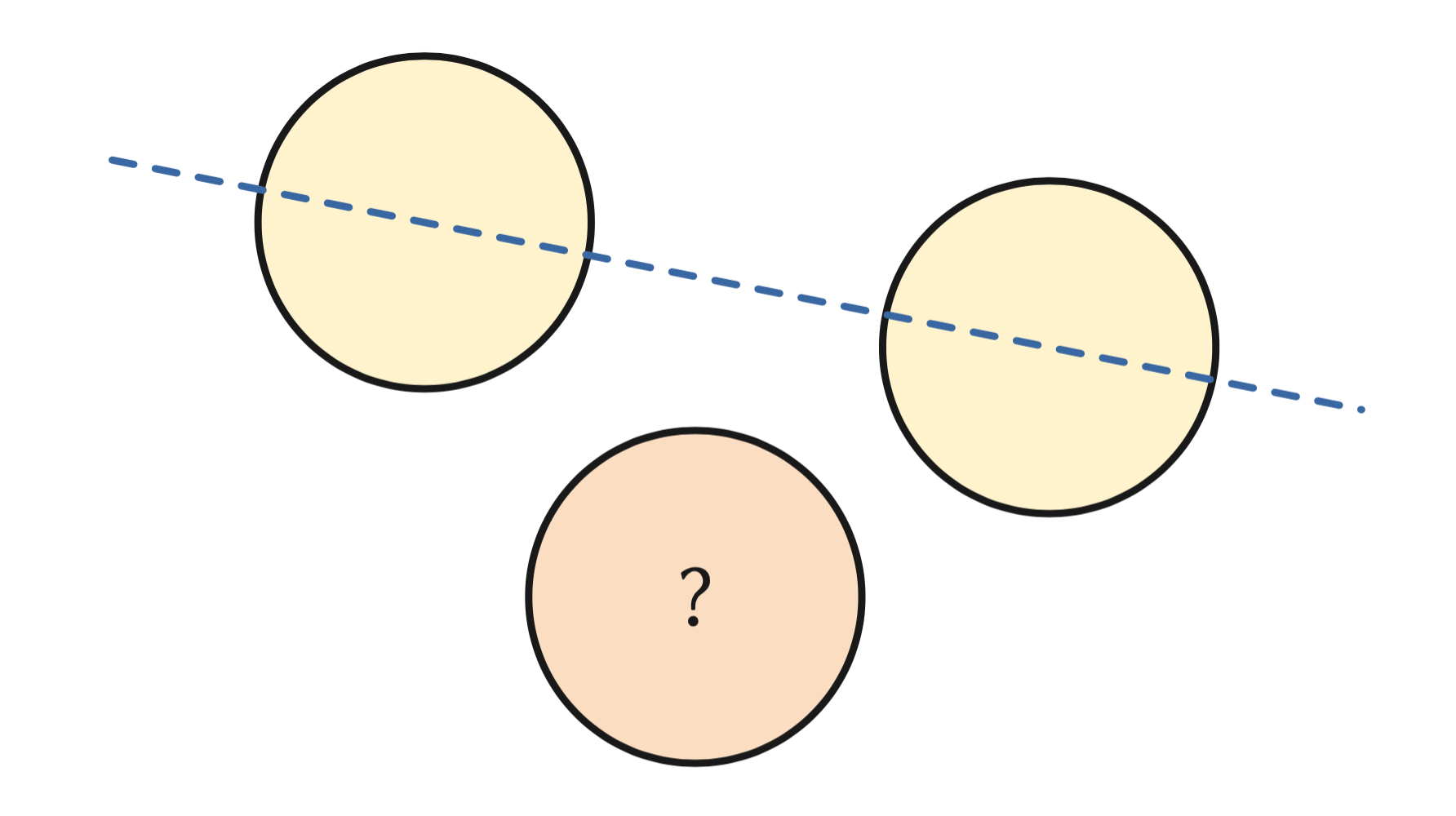It turns out the limit to this is the number of dimensions—in our two-dimensional space, we can take any two objects (of any size, shape or relative position) and find the unique line that cuts them both in half.

In three dimensions, we’d use a two-dimensional plane to cut things in half, but we could have three objects—in the classical case, two bits of bread and a piece of ham—such that we can find a 2D plane that cuts all three objects exactly in half. The objects can be arranged in any way you like, be different relative sizes and even intersect each other, and the ham sandwich theorem says this is still possible.It turns out this is generally true in $n$-dimensional space: using an $(n-1)$-dimensional slicing line or plane, we can cut $n$ objects exactly in half all at the same time. The calculations to determine exactly where that line or plane is might be a little more difficult, but the theorem guarantees that such a slice exists—so you can share your sandwich knowing that you’ve each got the same amount of bread and ham.

## The sausage catastrophe

Continuing with the food theme, I’ve always enjoyed the name of this particular geometrical occurrence, which concerns arrangements of spheres in different numbers of dimensions, and in particular the question of the surface area bounding a set of spheres.

Imagine a single sphere in 2D—a circle drawn on a page. The smallest region containing that circle is just the circle itself. But if I add a second circle touching it, in order to bound them I need to draw a shape which has rounded ends and straight edges—strictly called a stadium—whose perimeter will be the circumference of two half-circles, plus four times the radius of the circle:This kind of shape is called a convex hull—the smallest shape that encloses all the shapes and is mathematically convex. We can add in a third circle on the end of the line and draw a shape that encloses all three: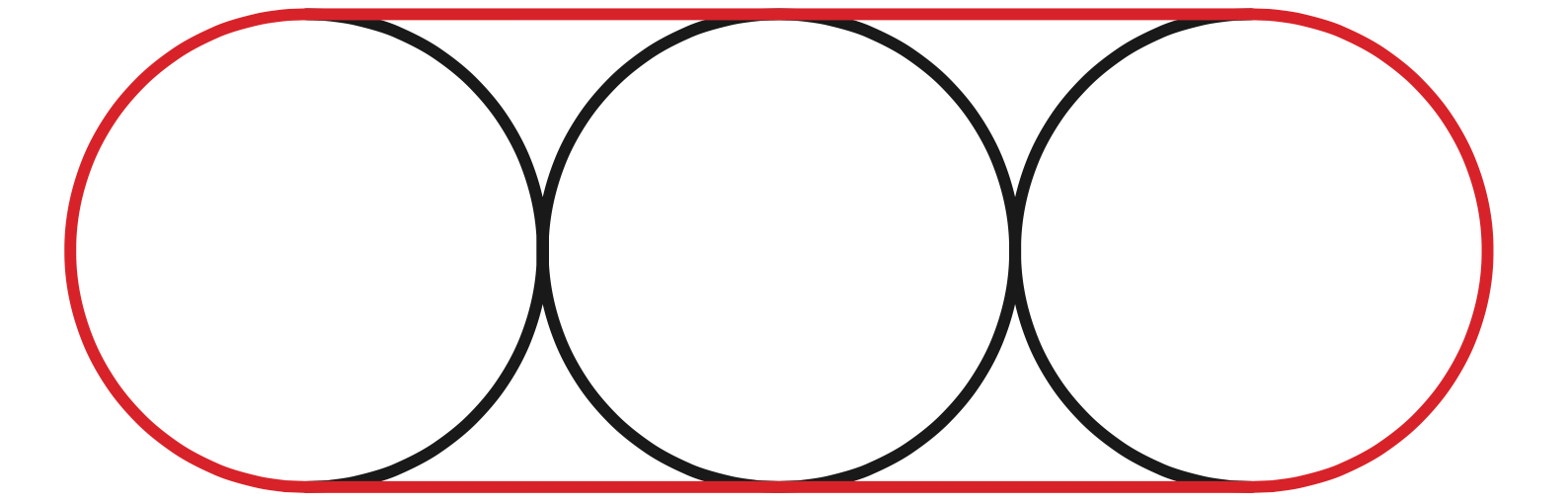but if we’re looking to minimise this surface area, we could maybe arrange the circles differently. If we put the three circles at the corners of an equilateral triangle so they’re all touching, maybe the perimeter of this shape is shorter?

Well, no. It turns out that for up to four circles (and up to four spheres, in higher dimensions) arranging the spheres into a long sausage gives the most efficient overall surface area…up to a point. For 2 dimensional circles, 3 dimensional spheres, 4D hyperspheres, and 5, 6, 7, 8,…dimensional whatever you call them above that, the sausage continues to be the most efficient shape all the way up to 55 dimensions. And then suddenly—it isn’t.For four 56-dimensional spheres, arranging them in a straight line doesn’t give the smallest surface area—there’s actually a different arrangement that gives a more efficient packing. For 57 and 58, it’s back to a sausage again. Then for 59: not a sausage. The most efficient arrangement continues to fluctuate (up to 65, beyond which sausage is always not the best) in a way that mathematicians found so distressing they named it the sausage catastrophe.

## The hairy ball theorem

No roundup of mathematical things with daft names would be complete without a mention of the most hilarious topological result, the hairy ball theorem. Despite being from the world of algebraic topology, which often comes with hefty dense terminology, it’s a surprisingly easy-to-understand concept.

A vector field, which consists of a vector pointing in a direction attached at every point of a space, can be thought of as a collection of tiny hairs attached to the surface (particularly if the space you’re considering is a two-dimensional surface like a manifold, or in this case, a sphere).

A ball with a hair attached at each point—a hairy ball, if you will—can be considered as a model for a sphere with a vector field. The conditions we require for the vector field to be well-behaved (ie continuous) are exactly those you’d get if the vectors were made out of real actual hairs with a thickness—they don’t lie exactly on top of each other, and move in the right kind of way—and you can manipulate the vector field by combing the hairs.

The theorem states that there is no continuous tangent vector field on even-dimensional $n$- spheres—that is to say, if we try to ‘comb’ the vector field so that all the vectors lie flat, we won’t be able to do this without at least one point where the hairs point directly upwards. You can see a failed attempt above right.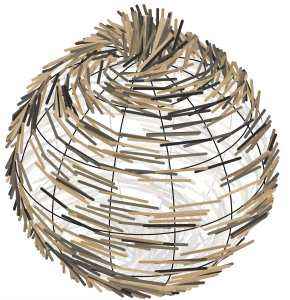A failed attempt to comb the hair on a ball flat

It’s famously the reason why the hair on a person’s head often forms a ‘cowlick’ (a bit at the crown of the head where it sticks up); it also has a nice consequence which is that at any given time, the vector field describing the movement of air on the Earth’s surface must have at least one point where it has no horizontal component to the motion—that is, there’s always somewhere on Earth where it isn’t currently windy.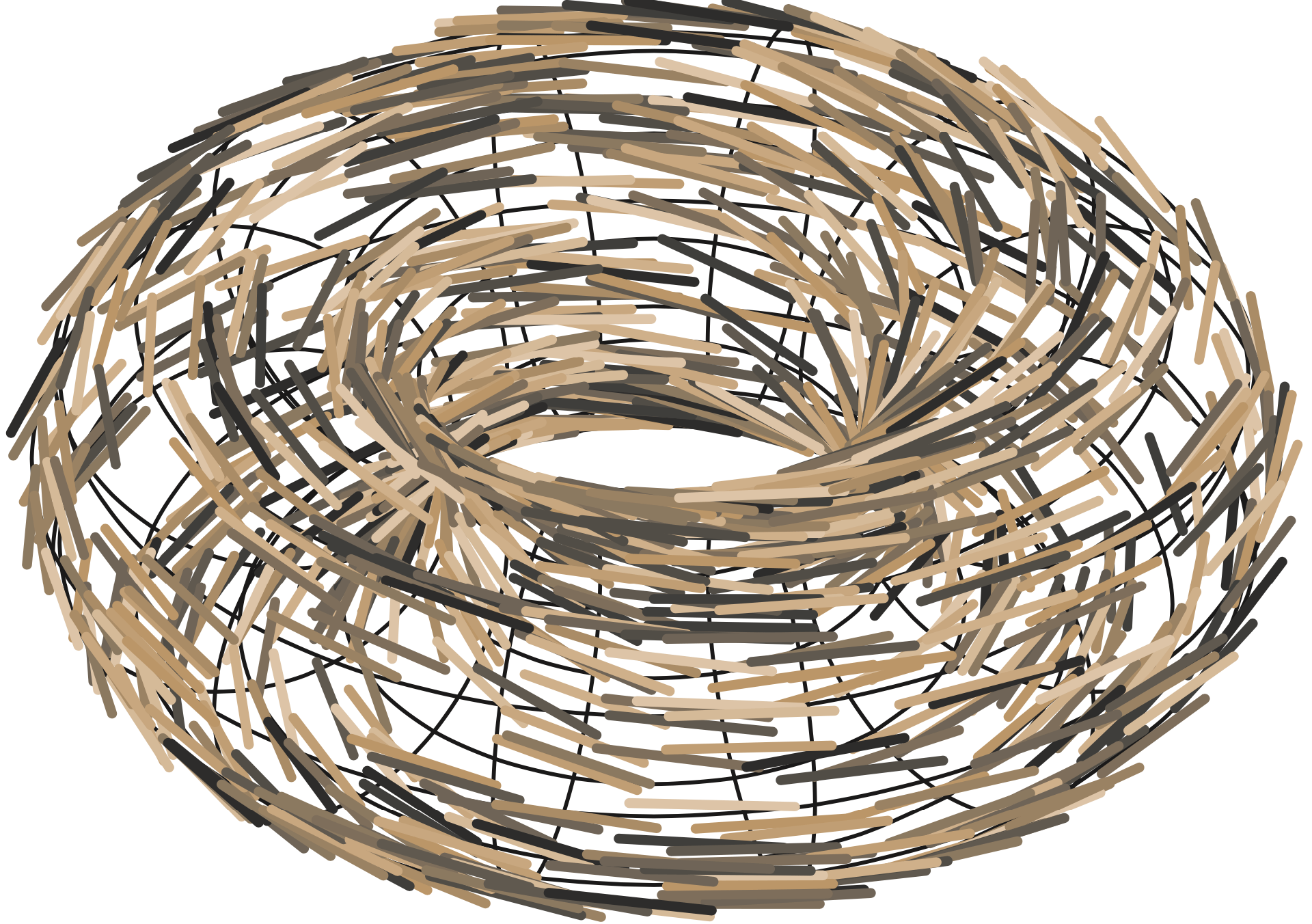A successful attempt to comb the hair on a torus flat

The theorem only applies in certain cases—the sphere needs to be even-dimensional (by dimension here we mean the number of dimensions its surface is made of, so a normal sphere sitting in 3D space is considered to be two-dimensional). It is possible to have a continuous tangent vector field on some shapes which aren’t spheres—for example, a 2-torus (doughnut) shape can be combed flat, by pointing all the hairs in the same direction: that of a circle running around the torus, like you can see above.

I’m sure there are many other mathematical theorems, constructions and ideas which have even sillier names than this—and I encourage you to find out about them and share them with as many people as you can. And, if you want a really good motivation for new mathematical research, consider the possibility that if you discover or prove a new piece of maths, you might even get to choose what it’s called.Katie Steckles is a mathematician based in Manchester, who gives talks and workshops on different areas of maths. She finished her PhD in 2011, and since then has talked about maths in schools, at science festivals, on BBC radio and TV, at music festivals, at comedy shows and on the internet. She blogs at the Aperiodical, and has a YouTube channel.